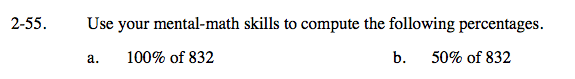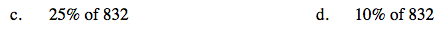### Home > CAAC > Chapter 2 > Lesson 2.1.5 > Problem2-55

2-55.
1. Use your mental-math skills to compute the following percentages. Homework Help ✎

1. 100% of 832

2. 50% of 832

3. 25% of 832

4. 10% of 832Convert the percent to a decimal.

Now multiply.

832

Follow the steps in part (a).

416Follow the steps in part (a).

83.2